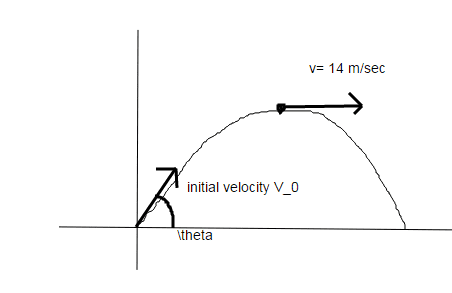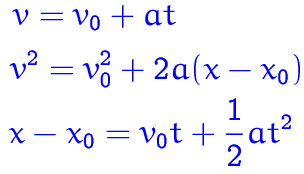# Kinematics velocity Question

## Homework Statement

The magnitude of the velocity of a projectile when it is at its maximum height above ground level is 14 m/s. (a) What is the magnitude of the velocity of the projectile 1.2 s before it achieves its maximum height? (b) What is the magnitude of the velocity of the projectile 1.2 s after it achieves its maximum height? If we take x = 0 and y = 0 to be at the point of maximum height and positive x to be in the direction of the velocity there, what are the (c) x coordinate and (d) y coordinate of the projectile 1.2 s before it reaches its maximum height and the (e) x coordinate and (f) y coordinate

## Homework Equations

Maybe will use component method

## The Attempt at a SolutionHave you tried anything yet?
- First time encountering this kind of problem, but by using my knowledge in vectors(which is not that big, so don't expect.) maybe I can say I'll use 14 as my y component and use some data (which I do not know) to get the x component. Then I can use it to get the hypotenuse or the magnitude.

- Well sorry if I lack information about this problem, well this is why I posted in this forum right? For someone to lend me a hand.

English is not my first language

Titan97
Gold Member
Does the x-component of velocity of projectile change during its motions?

Does the x-component of velocity of projectile change during its motions?

Well I think so. Yes. If I'm wrong, please tell me why, I would love to know. Thank you!

Titan97
Gold Member
What is the direction of acceleration of a projectile?

Titan97
Gold Member
Hint: What you said in post 3 is wrong

What is the direction of acceleration of a projectile?

Oh okay? I think it is up to down only so maybe the x-component will not change?

Then my drawing is wrong if that;s the case

Then my drawing is wrong if that;s the case
No , acceleration is in downward direction , but your diagram is still correct .

Titan97
Gold Member
Leave the diagram for now. Good. The acceleration is indeed along y-axis only. Now, write the kinematic equations for displacement of projectile along x and y-axis separately.
Take ux and uy as the initial velocity along x and y axis respectively and ax and ay as the acceleration. (You know the value of ax and ay)

Leave the diagram for now. Good. The acceleration is indeed along y-axis only. Now, write the kinematic equations for displacement of projectile along x and y-axis separately.
Take ux and uy as the initial velocity along x and y axis respectively and ax and ay as the acceleration. (You know the value of ax and ay)

I think you mean this?the 2nd equation I assume?

or you mean this
Vx = VoCosΘ
Vy = VoSinΘ

??

Titan97
Gold Member
Take the third kinematic equation (given in blue).
s=v0+½at2. Just write the equation along x and y axis separately.

Take the third kinematic equation (given in blue).
s=v0+½at2. Just write the equation along x and y axis separately.

Sooo okay
S=(14)+1/2(9.8)(1.2)^2
Am I right?
S=(14)+1/2(9.8)(-1.2)^2

The magnitude of the velocity of a projectile when it is at its maximum height above ground level is 14 m/s. (a) What is the magnitude of the velocity of the projectile 1.2 s before it achieves its maximum height? (b) What is the magnitude of the velocity of the projectile 1.2 s after it achieves its maximum height? If we take x = 0 and y = 0 to be at the point of maximum height and positive x to be in the direction of the velocity there, what are the (c) x coordinate and (d) y coordinate of the projectile 1.2 s before it reaches its maximum height and the (e) x coordinate and (f) y coordinate
You haven't done any problem of this sort before ? Have you not even read about it before ?

Projectile motion has different formulas , different conditions and you should read about it before trying any of it's questions . It isn't just a different kind of kinematics problem , it ( projectile motion ) consists of a whole set of different problems .

Last edited:
You haven't done any problem of this sort before ? Have you not even read about it before ?

Projectile motion has different formulas , different conditions and you should read about it before trying it's questions .

But I'm sorta getting the idea of freefall. I will change a into g right? 9.8 m/s^2

Titan97
Gold Member
You haven't done any problem of this sort before ? Have you not even read about it before ?

Projectile motion has different formulas , different conditions and you should read about it before trying it's questions .

I sort of understand free fall which lead to projectile motion right?
Look. I'm not totally clueless about the question. But a help is all I need, I'm not asking for answers.
I'm a type of person who understands lesson via examples.

This is my first time encountering this type of Q
but this is not my first time answering a free fall question.

Titan97
Gold Member
Please read about projectile motion and you will find that you could have solved the question yourself.If you are still not able to solve, then post your doubt on PF. I am helping you. I don't want to spoil the fun in solving this question.

Please read about projectile motion and you will find that you could have solved the question yourself. I am helping you. I don.t want to spoil the fun in solving this question.

Okay done reading. So can you guide me in the question?

Titan97
Gold Member
In the wikipedia page, the equation for displacement for projectile motion along x and y is given. Use that to find the time of flight. For that, substitute the value of y as zero since at the end of flight, the net displacement along y is zero.

Take the third kinematic equation (given in blue).
s=v0+½at2.
Please note typo. It should be s=v0t+1/2at2.

With (x,y)=(0,0) at the top of the curve, apply this equation to the x and y directions separately.

I got a and b last night. My answer for the magnitude is 18.2838

I got it from sqrt(14^2+11.76^2)

I got 11.76 from Vf = Vo-gt<- (9.8)(1.2)

soooo next is c,d,e,f.. any help?

what are the (c) x coordinate and (d) y coordinate of the projectile 1.2 s before it reaches its maximum height and the (e) x coordinate and (f) y coordinate
I assume this sentence should have ended "of the projectile 1.2 s after it reaches its maximum height."

First, the easy one: What's the x coordinate 1.2 s after max height? (What do we know about velocity in the x direction?)
Also, it helps to pick t=0 at the moment of max height.

berkeman
Mentor
I got a and b last night. My answer for the magnitude is 18.2838

I got it from sqrt(14^2+11.76^2)

I got 11.76 from Vf = Vo-gt<- (9.8)(1.2)

soooo next is c,d,e,f.. any help?

How about you keep going and post your work. We do not spoonfeed students here on the PF. We strive to help you how to learn here...

•Titan97
How about you keep going and post your work. We do not spoonfeed students here on the PF. We strive to help you how to learn here...

Idk what you mean by that? Bcccc I don't want to be spoonfed too. What I mean by help is to guide me? I read the guidelines about posting homeworks I think I've followed them. Like what I said english is not my first language so can you pls go easy on me will you? Nonetheless I've done finish the problem last night. So thank you I think